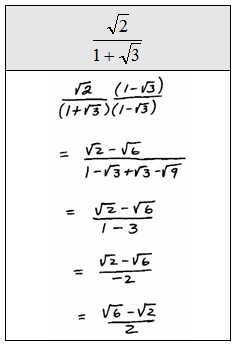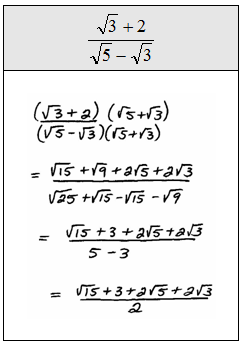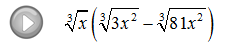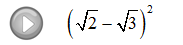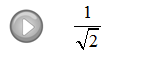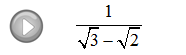## Algebra

Showing posts with label division. Show all posts
Showing posts with label division. Show all posts

### Synthetic Division

When dividing polynomials of the form p(x)/(x-a) we can use synthetic division as a shortcut for polynomial long division. Below we divide using traditional polynomial long division and synthetic division side by side.

Divide.
Both processes give the same result, x^2 - 3x - 2. However, synthetic division uses only the coefficients and requires much less writing.  To understand synthetic division, we walk you through the process below. Be sure the polynomials are in standard form, that is, each term is arranged in descending order from highest power to lowest.

Step 1: Write the root a determined from (x-a) and the coefficients of the polynomial in the first line.
Step 2: Bring down the first coefficient and we are ready to begin.
Step 3: Multiply a by the first coefficient and write the result under the second coefficient.
Step 4: Add the second column and write the result below.
Step 5: Repeat the process for all of the remaining columns.
Step 6: The numbers along the bottom are the coefficients of the result in standard form beginning with a term of degree one less than the original polynomial. The last number is the remainder.
To finish, clearly present the answer to your reader.  Next we do an example with a remainder. Just as we do in polynomial long division, we add a term that is the remainder over the divisor.

Divide.
Here the root (or zero) of (x+5)  is -5.
Multiplying both sides by the divisor (x+5) we have the following.
We mentioned that the polynomials are required to be in standard form. Sometimes there will be "missing terms."  That is, not all powers will have nonzero coefficients.  In this case, we use 0 as placeholders when performing synthetic division.

Divide.

Sometimes the root will be a fraction.

Divide.

It is interesting to note that the result has a GCF of 2 and we can do the following algebraic manipulations:
In short, this gives us a method of factoring a more complicated polynomial.

---

### Multiplying and Dividing Radical Expressions

As long as the indices are the same, we can multiply the radicands together using the following property.Since multiplication is commutative, you can multiply the coefficients and the radicands together and then simplify.

Multiply.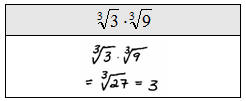Take care to be sure that the indices are the same before multiplying.  We will assume that all variables are positive.

Simplify.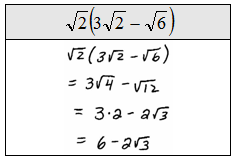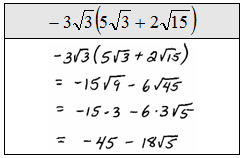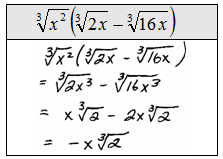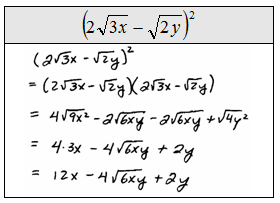Divide radicals using the following property.Divide. (Assume all variables are positive.)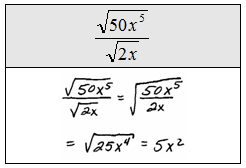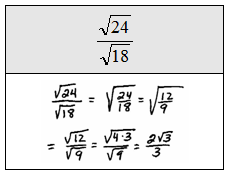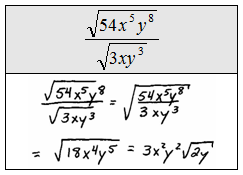Rationalizing the Denominator
A simplified radical expression cannot have a radical in the denominator.  When the denominator has a radical in it, we must multiply the entire expression by some form of 1 to eliminate it.  The basic steps follow.

Rationalize the denominator: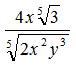Multiply numerator and denominator by the 5th root of of factors that will result in 5th powers of each factor in the radicand of the denominator.Notice that all the factors in the radicand of the denominator have powers that match the index.  This was the desired result, now simplify.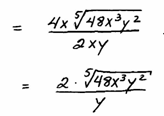Rationalize the denominator.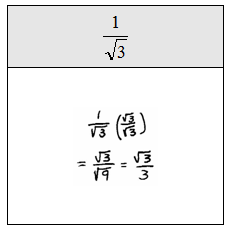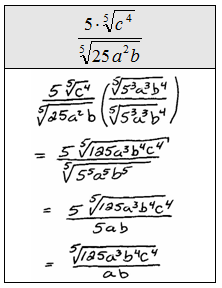This technique does not work when dividing by a binomial containing a radical.  A new technique is introduced to deal with this situation.

Rationalize the denominator:Multiply numerator and denominator by the conjugate of the denominator.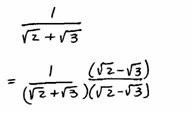And then simplify. The goal is to eliminate all radicals from the denominator.Rationalize the denominator.